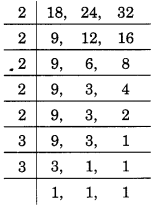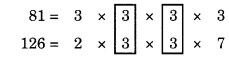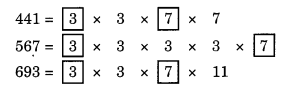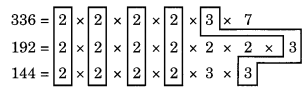# Playing With Numbers Class 6 Extra Questions Maths Chapter 3

Playing With Numbers Class 6 Extra Questions Maths Chapter 3

### Playing With Numbers Class 6 Extra Questions Maths Chapter 3

Extra Questions for Class 6 Maths Chapter 3 Playing With Numbers

### Playing With Numbers Class 6 Extra Questions Very Short Answer Type

Question 1.
What is the sum of any two
(a) even numbers
(b) odd numbers?
Solution:
(a) The sum of any two even numbers is even.
Example: 4 (even) + 6 (even) = 10 (even)
(b) The sum of any two odd numbers is even.
Example: 5 (odd) + 7 (odd) = 12 (even)

Question 2.
Which of the following numbers is divisible by 3?
(a) 1212
(b) 625
Solution:
(a) Given number = 1212
Sum of the digits = 1 + 2 + 1 + 2 = 6, which is divisible by 3.
Hence, 1212 is also divisible by 3.
(b) Given number = 625
Sum of the digits = 6 + 2 + 5 = 13, which is not divisible by 3.
Hence, 625 is not divisible by 3.

Question 3.
If the LCM and HCF of any two numbers are 15 and 4 respectively, find the product of the numbers.
Solution:
We know that the product of the number = LCM x HCF = 15 x 4 = 60
Hence, the product of the given numbers = 60.

Question 4.
Find the HCF of 5 and 7.
Solution:
Given numbers are 5 and 7. We observe that 5 and 7 are co-prime numbers.
Hence, the HCF is 1.

Question 5.
Write first 3 multiples of 25.
Solution:
We have 25 x 1 = 25; 25 x 2 = 50; 25 x 3 = 75
Hence, the required multiples are 25, 50 and 75.

Question 6.
What are the possible factors of (a) 12 (b) 18?
Solution:
(a) Possible factors of 12 are:
12 = 1 x 12; 12 = 2 x 6; 12 = 3 x 4
Hence, the factors of 12 are 1, 2, 3, 4, 6 and 12.

(b) Possible factors of 18 are:
18 = 1 x 18; 18 = 2 x 9; 18 = 3 x 6
Hence, the factors of 18 are 1, 2, 3, 6, 9 and 18.

Question 7.
Write first three multiples of 11.
Solution:
First three multiples of 11 are:
11 x 1 = 11; 11 x 2 = 22; 11 x 3 = 33
Hence, the required multiples are: 11,22 and 33.

Question 8.
Write pairs of twin prime numbers less than 20.
Solution:
Pairs of twin prime numbers are: (3, 5), (5, 7), (11, 13), (17, 19).

Question 9.
Write the number which is even as well as prime.
Solution:
2 is the only even number which is prime number also.

Question 10.
What is the fundamental theorem of arithmetic?
Solution:
Every number greater than 1 has exactly one prime factorisation.

### Playing With Numbers Class 6 Extra Questions Short Answer Type

Question 11.
Simplify: 32 + 96 ÷ (7 + 9)
Solution:
Given that: 32 + 96 ÷ (7 + 9)
= 32 + 96 ÷ 16 (Using BODMAS)
= 32 + 6 = 38

Question 12.
Simplify: 18 + {1 + (5 – 3) x 5}
Solution:
Given that: 18 + {1 + (5 – 3) x 5} (Using BODMAS)
= 18 + {1 + 2 x 5} = 18 + {1 + 10}
= 18 + 11 = 29.

Question 13.
Without actual division, show that 11 is a factor of 1,10,011.
Solution:
Here 1,10,011 = 1,10,000 + 11
= 11 x 10,000 + 11 x 1
= 11 x (10,000 + 1)
= 11 x 10,001
It is clear that 11 is a factor of 11 x 10,001.
Hence, 11 is a factor of 1,10,011.

Question 14.
The sum of two numbers is 25 and their product is 144. Find the numbers.
Solution:
The product of two numbers is 144.
∴ The possible factors are 1 x 144, 2 x 72, 3 x 48, 4 x 36, 6 x 24, 8 x 18, 9 x 16, 12 x 12
Here, we observe that out of these factors, we take 9 and 16.
Product = 9 x 16 = 144 and sum = 9 + 16 = 25
Hence, the required numbers are 9 and 16.

Question 15.
Is 80136 divisible by 11?
Solution:
Sum of the digits at odd places = 6 + 1 + 8 = 15
Sum of the digits at even places = 3 + 0 = 3
Difference of the two sums = 15 – 3 = 12,
which is neither 0 nor the multiple of 11.
Hence, 80136 is not divisible by 11.

Question 16.
The HCF and LCM of two numbers are 6 and 120 respectively. If one of the numbers is 24, find the other number.
Solution:
Given that: HCF = 6
LCM = 120
Let the two numbers be a and b, where a = 24, b = ?
We know that: a x b = HCF x LCM
⇒ 24 x b = 6 x 120
⇒ b = 6x120/24
⇒ b = 30
Hence, the other number is 30.

Question 17.
Find the LCM of 12 and 30.
Solution:
Given numbers are 12 and 30
12 = 2 x 2 x 3;
30 = 2 x 3 x 5
∴ LCM = 2 x 2 x 3 x 5 = 60
Hence, the LCM of 12 and 30 = 60.

Question 18.
Find the smallest 4-digit number which is divisible by 18, 24 and 32.
Solution:
Given numbers are 18, 24 and 32, we haveThus, LCM = 2 x 2 x 2 x 2 x 2 x 3 x 3 = 288
The smallest 4-digit number = 1000
Now, we write multiples of 288, till we get a 4-digit number.
288 x 1 = 288, 288 x 2 = 576,
288 x 3 = 864, 288 x 4 = 1152
Hence, 1152 is the required number.

Question 19.
Find the greatest number which divides 82 and 132 leaving 1 and 6, respectively as remainders.
Solution:
Given numbers are 82 and 132 and the remainders are 1 and 6 respectively.
We have, 82 – 1 = 81 and 132 – 6 = 126
So, we need to find the HCF of 81 and 126Common factor is 3 (occurring twice).
∴ HCF = 3 x 3 = 9
Hence, the required number is 9.

Question 20.
Find the greatest number that will divide 455, 582 and 710 leaving remainders 14, 15 and 17 respectively.
Solution:
Given numbers are 455, 582 and 710 and the respective remainders are 14, 15 and 17.
We have 455 – 14 = 441, 582 – 15 = 567 and 710 – 17 = 693.
Now let us find their HCF.Common factors are 3 and 7.
∴ HCF = 3 x 7 = 21
Hence, the required number is 21.

### Playing With Numbers Class 6 Extra Questions Long Answer Type

Question 21.
Simplify the following:
40 + [20 – {28 ÷ 7 – 3 + (30 – 5 of 4)}]
Solution:
Using BODMAS Rule, we have
40 + [20 – {28 ÷ 7 – 3 + (30 – 5 of 4)}]
= 40 + [20 – {28 ÷ 7 – 3 + (30 – 20)}]
= 40 + [20 – {28 ÷ 7 – 3 + 10}]
= 40 + [20 – [4 – 3 + 10}]
= 40 + [20-11] = 40 + 9 = 49.

Question 22.
Three sets of English, Hindi, and Urdu books are to be stacked in such a way that the books are stored subjectwise and the height of each stack is the same. The numbers of English, Hindi and Urdu books are 336, 192 and 144 respectively. Assuring that the books have that same thickness, determine the number of stacks of English, Hindi and Urdu books.
Solution:
To arrange the books in the required way,
we have to find the greatest number that divides 336, 192 and 144 exactly.
So, HCF of 336, 192 and 144 isCommon factors are 2 x 2 x 2 x 2 x 3 = 48
∴ HCF = 48, i.e. each stack contains 48 books.
∴ Number of stacks of English books = 336 + 48 = 7
Number of stacks of Hindi books = 192 4- 48 = 4
Number of stacks of Urdu books = 144 4- 48 = 3

Question 23.
Which of the following statements are true?
(а) 1371 is divisible by 3
(b) 1155 is not divisible by 9
(c) 1478 is not divisible by 4
(d) 2470 is divisible by 5
(e) If a number is divisible by 9, it is also divisible by 3.
(f) If a number is divisible by 3, it is also divisible by 9.
(g) The sum of any two odd numbers is even.
(h) If a number is divisible by 8, it must be divisible by 6.
(i) If a number is divisible by 3 and 6, it is divisible by 18.
(j) 1758 is not divisible by 8.
Solution:
(a) Yes, 1371 is divisible by 3. So it is true statement.
(b) Yes, 1155 is not divisible by 9. So it is true statement.
(c) Yes, 1478 is not divisible by 4. So it is true statement.
(d) Yes, 2470 is divisible by 5. So it is true statement.
(e) Yes, it is true statement. if No, it is not true statement.
(g) Yes, it is true statement.
(h) No, it is not true statement.
(i) No, it is not true statement.
(j) Yes, it is true statement.

### Playing With Numbers Class 6 Extra Questions Multiple Choice Type

Question 24.
Which of the following numbers is divisible by 11?
(a)112111
(b) 928389
(c) 12011
(d) 11111

Question 25.
Match column I with column II.
Column I                                                                               Column II
(a) A number divisible by 11                                                     (i) 2
(b) HCF of two consecutive odd numbers                            (ii) 4
(c) The difference between twin prime number                 (iii) product of the number
(d) Number of factors of a prime number                            (iv) 60
(e) Lowest composite number                                                 (v)2
(f) LCM of 12 and 5                                                                    (vi) 4587594
(g) The smallest prime number                                              (vii) 1
(h) Product of HCF and LCM is equal to                              (viii) 2
Solution:
(a) → (vi)
(b) → (vii)
(c) → (viii)
(d) → (i)
(e) → (ii)
(f) → (iv)
(g) → (v)
(h) → (iii)

### Playing With Numbers Class 6 Extra Questions Higher Order Thinking Skills (HOTS)

Question 26.
Ill cows, 185 sheep and 296 goats are to be taken across a river. There is only one boat and the boatsman says; he will take the same number and same kind of animals in each trip. Find the largest number of animals in each trip and the number of trips he will have to make.
Solution:
We have
Number of cows = 111
Number of sheep = 185
Number of goats = 296
According to the condition of the boatsman, we need to find HCF of 111, 185 and 296
111 = 3 x 37;
185 = 5 x 37;
296 = 2 x 2 x 2 x 37
∴ the HCF = 37
So, the number of animals of same kind = 37.
Number of trips
111/37 + 185/37 + 296/37
= 3 + 5 + 8 = 16
Hence, the number of animals in each trip = 37
and the number of trips = 16.

Question 27.
In a seminar, the number of participants in Mathematics, Physics and Chemistry are 60, 96 and 144 respectively. Find the number of rooms required if in each room, the same number of ‘ participants are to be seated and all of them are to be in the same subject.
Solution:
The number of participants in each room must be the HCF of 60, 96 and 144.
∴ 60 = 2 x 2 x 3 x 5
96 = 2 x 2 x 2 x 2 x 2 x 3
144 = 2 x 2 x 2 x 2 x 3 x 3
HCF = 2 x 2 x 3 = 12
Number of rooms required
60/12 + 96/12 + 144/12
= 5 + 8 + 12 = 25
Hence, number of participants = 12
in each room and number of rooms required = 25.

## SabDekho

The Complete Educational Website